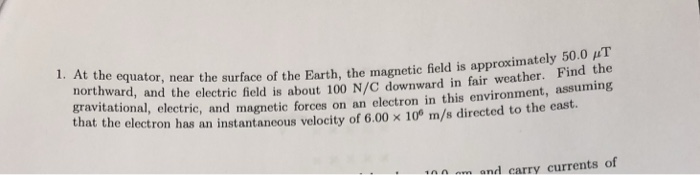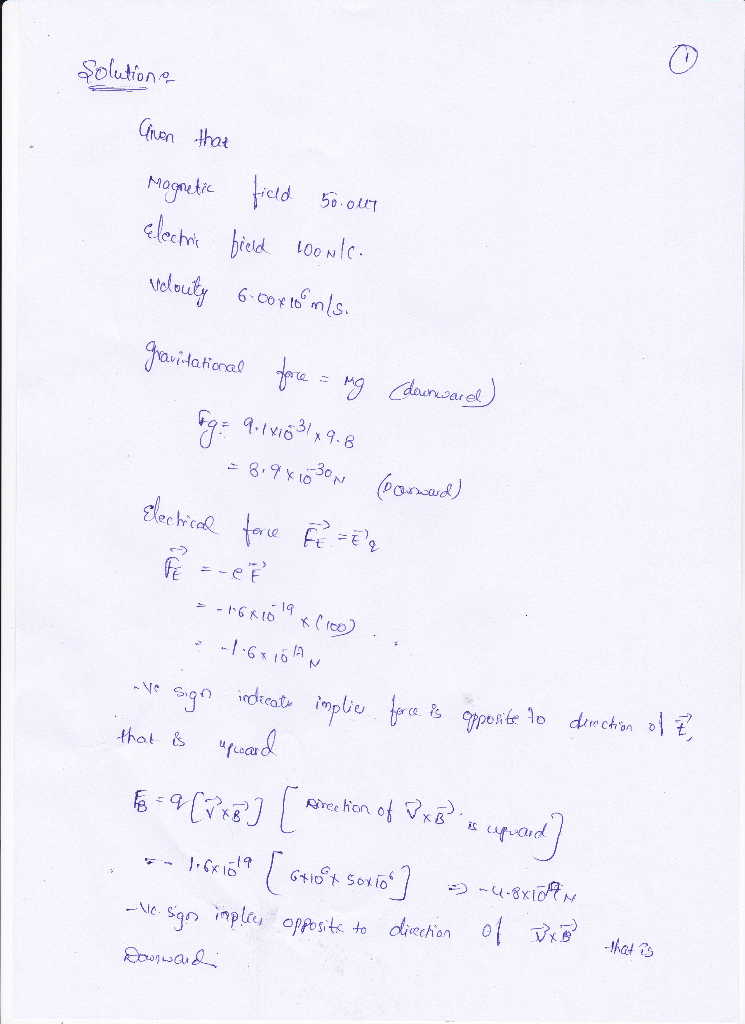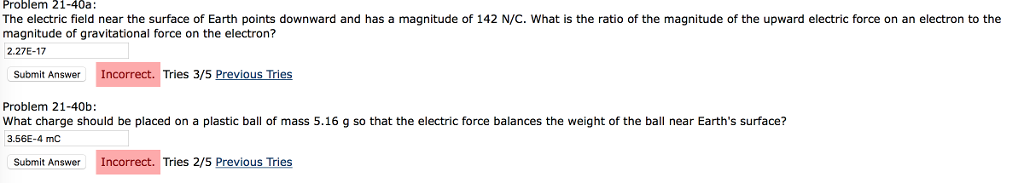# 50.0 HT Find the 1. At t e equator, near the surface of the Earth, the...50.0 HT Find the 1. At t e equator, near the surface of the Earth, the magnetic field is approximately rward, and the electric field is about 100 N/C downward in fair weather. F avitational, electric, and magnetic forces on an electron in this environment, assuming that the elect ron has an instantaneous velocity of 6.00 × 106 m/s directed to the east. to n am and carry currents of#### Earn Coin

Coins can be redeemed for fabulous gifts.

Similar Homework Help Questions
• ### The electric field near the surface of Earth points downward and has a magnitude of 152...

The electric field near the surface of Earth points downward and has a magnitude of 152 N/C. What is the ratio of the magnitude of the upward electric force on an electron to the magnitude of gravitational force on the electron? What charge should be placed on a plastic ball of mass 4.06 g so that the electric force balances the weight of the ball near Earth's surface?

• ### The electric field near the surface of Earth points downward and has a magnitude of 148...

The electric field near the surface of Earth points downward and has a magnitude of 148 N/C. What is the ratio of the magnitude of the upward electric force on an electron to the magnitude of gravitational force on the electron? Problem 21-40b: What charge should be placed on a plastic ball of mass 5.38 g so that the electric force balances the weight of the ball near Earth's surface?

• ### The electric field near the surface of Earth points downward and has a magnitude of 148...

The electric field near the surface of Earth points downward and has a magnitude of 148 N/C. What is the ratio of the magnitude of the upward electric force on an electron to the magnitude of gravitational force on the electron? Tries 0/5 Problem 21-40b: What charge should be placed on a plastic ball of mass 5.38 g so that the electric force balances the weight of the ball near Earth's surface?

• ### Near the surface of Earth an electric field points radially downward and has a magnitude of...

Near the surface of Earth an electric field points radially downward and has a magnitude of approximately 100 N/C. What charge (magnitude and sign) would have to be placed on a penny that has a mass of 3 g to cause it to rise into the air with an upward acceleration of 0.19 m/s2?

• ### Near the surface of the Earth, an electric field points radially downward and has a magnitude...

Near the surface of the Earth, an electric field points radially downward and has a magnitude of approximately 100 N/C. What charge (magnitude and sign) would have to be placed on a penny that has a mass of 3.11 g to cause it to rise into the air with an upward acceleration of 0.19 m/s^2?

• ### Near the surface of Earth an electric field points radially downward and has a magnitude of...

Near the surface of Earth an electric field points radially downward and has a magnitude of approximately 100 N/C. What charge (magnitude and sign) would have to be placed on a penny that has a mass of 3.11 g to cause it to rise into the air with an upward acceleration of 0.190 m/s2? PLEASE SHOW DIAGRAM AND EACH ALGEBRAIC STEPS.

• ### Near the surface of Earth, an electric field points radially downward and has a magnitude of...

Near the surface of Earth, an electric field points radially downward and has a magnitude of approximately 100 N/C. 1) What charge in mC (magnitude and sign) would have to be placed on a penny that has a mass of 3.11 g to cause it to rise into the air with an upward acceleration of 1.990 m/s2? (Express your answer to three significant figures.)

• ### Ch.25 Question 48

In fair weather, the electric field in the air at a particularlocation immediately above the Earth's surface is 101 N/C directed downward. (a) What is thesurfacecharge density on the ground?1(b) Imagine the atmosphere is stripped off and the surface chargedensity is uniform over the planet. What then is the charge of thewhole surface of the Earth?(c) What is the Earth's electric potential?(d) What is the difference in potential between the head and thefeet of a person 1.60 m tall?(e) Imagine...

• ### Problem 21-40a: The electric field near the surface of Earth points downward and has a magnitude...Problem 21-40a: The electric field near the surface of Earth points downward and has a magnitude of 142 N/C. What is the ratio of the magnitude of the upward electric force on an electron to the magnitude of gravitational force on the electron? 2.27E-17 Submit Answer Incorrect. Tries 3/5 Previous Tries Problem 21-40b What charge should be placed on a plastic ball of mass 5.16 g so that the electric force balances the weight of the ball near Earth's surface?...

• ### Near the surface of Earth, an electric field points radially downward and has a magnitude of...

Near the surface of Earth, an electric field points radially downward and has a magnitude of approximately 100 N/C. What charge (magnitude and sign) would have to be placed on a penny that has a mass of 3.11 g to cause it to rise into the air with an upward acceleration of 0.590 m/s2? (Express your answer to three significant figures.) I keep getting 3.23 or some variation of that but it is not correct. Please show your work!